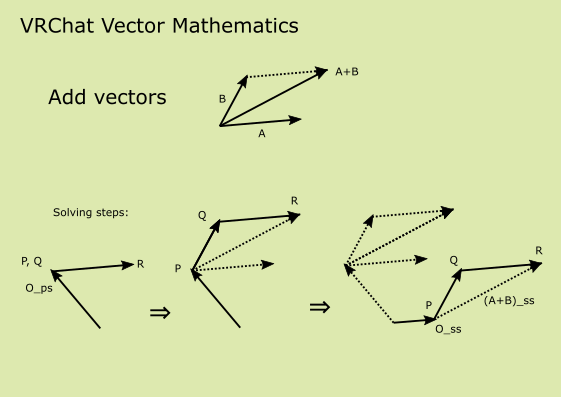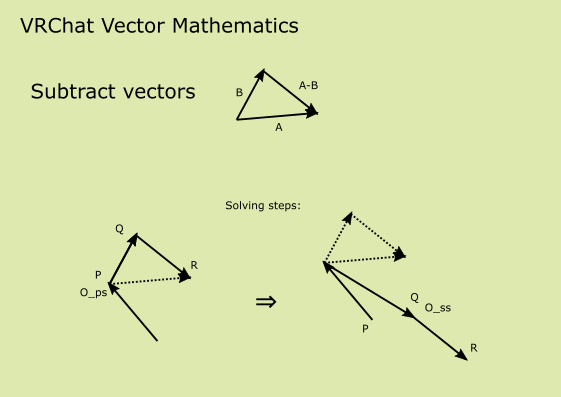# VRChat Vector Mathematics

Ways to calculate vector with GameObject transform and VRC_SceneResetPosition.

Object transform and VRC_SceneResetPosition are vector math library in VRChat!

## Notation

• For any vectors : `A`, `B`
• For local space origin : `O`
• For rotation matrix : `r`
• For scalar : `k`
• For indexing local space :
• `_ps`: in problem space
• `_ss`: in solution space

Object hierarchy :

``````P        // local root
- Q     // child object
- R  // grand child object
``````

## Move vector

Move a vector to another local space. `A_ps` => `A_ss`

implementation:

1. Move `P` to `O_ps`
2. Move `Q` to `A_ps`
3. Move `P` to `O_ss`
4. Then, `Q` is at `A_ss`

Add two vectors in problem space and get result in solution space. ( `A_ps`, `B_ps` ) => `(A + B)_ss`implementation:

1. Move `P` and `Q` to `O_ps`
2. Move `R` to `A_ps`
3. Move `Q` to `B_ps`
4. Move `P` to `O_ss` (skippable if `O_ps = O_ss`)
5. Then, `R` is at `(A + B)_ss`

## Subtract vectors

Subtract two vectors in problem space and get result in solution space. ( `A_ps`, `B_ps` ) => `(A - B)_ss`implementation:

1. Move `P` to `O_ps`
2. Move `Q` to `B_ps`
3. Move `R` to `A_ps` (`R`'s local transform is subtract result)
4. Move `Q` to `O_ss`
5. Then, `R` is at `(A - B)_ss`

## Rotate and scalar multiplication

Rotate a vectors in problem space and get result in solution space. Scalar multiplication solution is identical. So both could be done at once.

• ( `r_ps`, `A_ps` ) => `(r * A)_ss`
• ( `k`, `A_ps` ) => `(k * A)_ss`
• ( `k`, `r_ps`, `A_ps` ) => `(k * r * A)_ss`

implementation:

1. Move `P` and `Q` to `O_ps`
2. Move `R` to `A_ps`
3. Move `Q` to `r_ps`
4. Move `P` to `O_ss`
5. Then, `R` is at `(r * A)_ss`

## Acknowledgments

I lean this technique from http://vrchat.wikidot.com/worlds:guides:player-tracking by CyanLaser. Thank you for sharing your wonderful work.